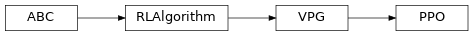# `garage.torch.algos.ppo`¶

Proximal Policy Optimization (PPO).

class PPO(env_spec, policy, value_function, sampler, policy_optimizer=None, vf_optimizer=None, lr_clip_range=0.2, num_train_per_epoch=1, discount=0.99, gae_lambda=0.97, center_adv=True, positive_adv=False, policy_ent_coeff=0.0, use_softplus_entropy=False, stop_entropy_gradient=False, entropy_method='no_entropy')Proximal Policy Optimization (PPO).

Parameters
• env_spec (EnvSpec) – Environment specification.

• policy (garage.torch.policies.Policy) – Policy.

• value_function (garage.torch.value_functions.ValueFunction) – The value function.

• sampler (garage.sampler.Sampler) – Sampler.

• policy_optimizer (garage.torch.optimizer.OptimizerWrapper) – Optimizer for policy.

• vf_optimizer (garage.torch.optimizer.OptimizerWrapper) – Optimizer for value function.

• lr_clip_range (float) – The limit on the likelihood ratio between policies.

• num_train_per_epoch (int) – Number of train_once calls per epoch.

• discount (float) – Discount.

• gae_lambda (float) – Lambda used for generalized advantage estimation.

• center_adv (bool) – Whether to rescale the advantages so that they have mean 0 and standard deviation 1.

• positive_adv (bool) – Whether to shift the advantages so that they are always positive. When used in conjunction with center_adv the advantages will be standardized before shifting.

• policy_ent_coeff (float) – The coefficient of the policy entropy. Setting it to zero would mean no entropy regularization.

• use_softplus_entropy (bool) – Whether to estimate the softmax distribution of the entropy to prevent the entropy from being negative.

• entropy_method (str) – A string from: ‘max’, ‘regularized’, ‘no_entropy’. The type of entropy method to use. ‘max’ adds the dense entropy to the reward for each time step. ‘regularized’ adds the mean entropy to the surrogate objective. See https://arxiv.org/abs/1805.00909 for more details.

property discount

Discount factor used by the algorithm.

Returns

discount factor.

Return type

float

train(trainer)

Obtain samplers and start actual training for each epoch.

Parameters

trainer (Trainer) – Gives the algorithm the access to :method:`~Trainer.step_epochs()`, which provides services such as snapshotting and sampler control.

Returns

The average return in last epoch cycle.

Return type

float